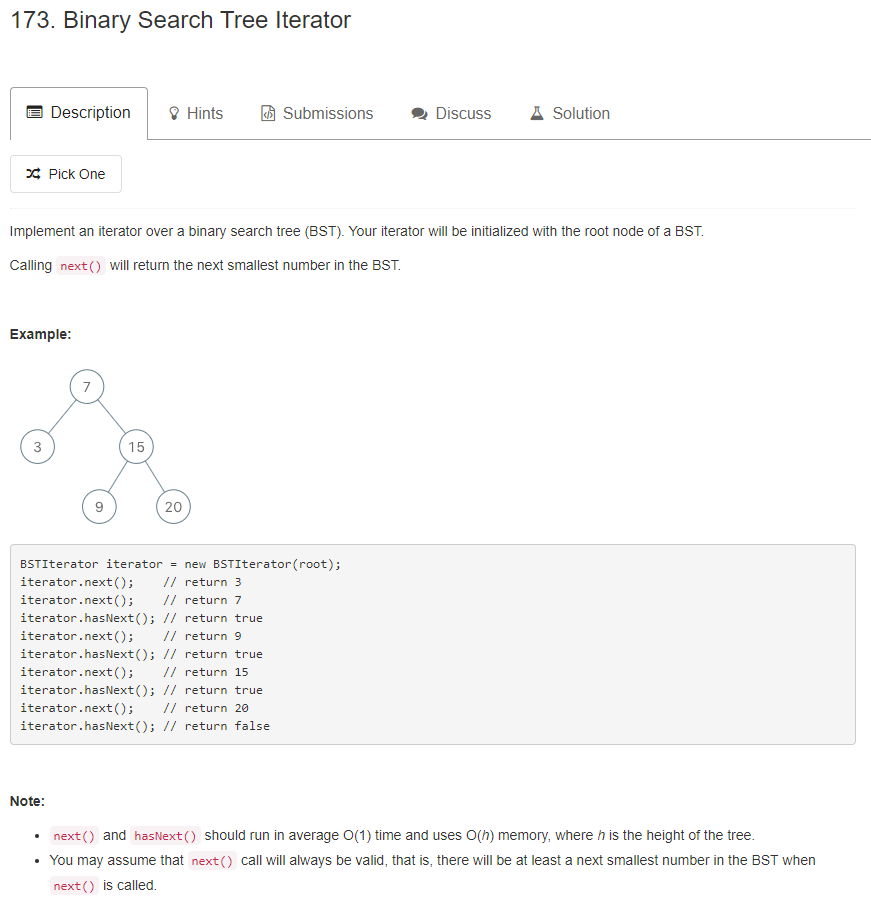# 题目描述（中等难度）1. 若任意节点的左子树不空，则左子树上所有节点的值均小于它的根节点的值；
2. 若任意节点的右子树不空，则右子树上所有节点的值均大于它的根节点的值；
3. 任意节点的左、右子树也分别为二叉查找树；
4. 没有键值相等的节点。

# 解法一

class BSTIterator {

public BSTIterator(TreeNode root) {
inorderTraversal(root);
}

private void inorderTraversal(TreeNode root) {
if (root == null) {
return;
}
inorderTraversal(root.left);
queue.offer(root.val);
inorderTraversal(root.right);
}

/** @return the next smallest number */
public int next() {
return queue.poll();
}

/** @return whether we have a next smallest number */
public boolean hasNext() {
return !queue.isEmpty();
}
}


# 解法二

public List<Integer> inorderTraversal(TreeNode root) {
List<Integer> ans = new ArrayList<>();
Stack<TreeNode> stack = new Stack<>();
TreeNode cur = root;
while (cur != null || !stack.isEmpty()) {
//节点不为空一直压栈
while (cur != null) {
stack.push(cur);
cur = cur.left; //考虑左子树
}
//节点为空，就出栈
cur = stack.pop();
//当前值加入
//考虑右子树
cur = cur.right;
}
return ans;
}


class BSTIterator {
Stack<TreeNode> stack = new Stack<>();
TreeNode cur = null;

public BSTIterator(TreeNode root) {
cur = root;
}

/** @return the next smallest number */
public int next() {
int res = -1;
while (cur != null || !stack.isEmpty()) {
// 节点不为空一直压栈
while (cur != null) {
stack.push(cur);
cur = cur.left; // 考虑左子树
}
// 节点为空，就出栈
cur = stack.pop();
res = cur.val;
// 考虑右子树
cur = cur.right;
break;
}

return res;
}

/** @return whether we have a next smallest number */
public boolean hasNext() {
return cur != null || !stack.isEmpty();
}
}